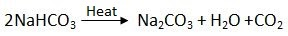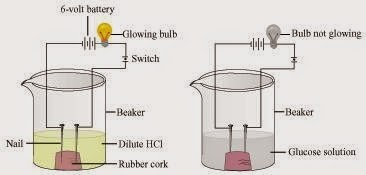## Breaking

CustomWritings.com - get an essay written for you by academic experts. Feel free to get writing assistance online.

## NCERT Solutions for Class 10 Science Chapter 2 – Acids, Bases and Salts

In Text Questions

Page No: 18

1. You have been provided with three test tubes. One of them contains distilled water and the other two contain an acidic solution and a basic solution, respectively. If you are given only red litmus paper, how will you identify the contents of each test tube?

If the colour of red litmus does not change then it is acid. If the colour of red litmus changes to blue then it is base. If there is slight change in the colour of red litmus (such as purple) then it is distilled water.

Page No: 22

1. Why should curd and sour substances not be kept in brass and copper vessels?

Curd and other sour substances contain acids. Therefore, when they are kept in brass and copper vessels, the metal reacts with the acid to liberate hydrogen gas and harmful products, thereby spoiling the food.

2. Which gas is usually liberated when an acid reacts with a metal? Illustrate with an example. How will you test for the presence of this gas?

Hydrogen gas is usually liberated when an acid reacts with metal.Take few pieces of zinc granules and add 5 ml of dilute H2SO4. Shake it and pass the gas produced into a soap solution. The bubbles of the soap solution are formed. These soap bubbles contain hydrogen gas.
H2SO4 + Zn → ZnSO4 + H2 ↑
We can test the evolved hydrogen gas by its burning with a pop sound when a candle is brought near the soap bubbles.

3. Metal compound A reacts with dilute hydrochloric acid to produce effervescence. The gas evolved extinguishes a burning candle. Write a balanced chemical equation for the reaction if one of the compounds formed is calcium chloride.

CaCO3 (s) + 2HCl (aq) → CaCl2 (aq) + CO2 (g) + H2O (l)
Calcium Carbonate + Hydrochloric acid → Calcium Chloride + Carbon dioxide + Water

Page No: 25

1. Why do HCl, HNO3, etc., show acidic characters in aqueous solutions while solutions of compounds like alcohol and glucose do not show acidic character?

When HCl or HNO3 are mixed with water then they dissolve in water to form H+ or H3O+ ions which shows their acidic character. For example just see the following reactions

HCl (aq) → H+ + Cl-
H+ + H2O → H3O+
When alcohols and glucose are mixed with water then they do not dissolve to form ions. Hence they do not show acidic character.

2. Why does an aqueous solution of an acid conduct electricity?

The presence of hydrogen (H+) or hydronium (H3O+) ions in the aqueous solution of an acid are responsible for conducting electricity.

3. Why does dry HCl gas not change the colour of the dry litmus paper?

Dry HCl gas not change the colour of the dry litmus paper because it has no Hydrogen ions (H+) in it.

4. While diluting an acid, why is it recommended that the acid should be added to water and not water to the acid?

Since the process of dissolving an acid in water is exothermic, it is always recommended that acid should be added to water. If it is done the other way, then it is possible that because of the large amount of heat generated, the mixture splashes out and causes burns.

5. How is the concentration of hydronium ions (H3O+) affected when a solution of an acid is diluted?

When an acid is diluted, the concentration of hydronium ions (H3O+) per unit volume decreases. This means that the strength of the acid decreases.

6. How is the concentration of hydroxide ions (OH) affected when excess base is dissolved in a solution of sodium hydroxide?

The concentration of hydroxide ions (OH) would increase when excess base is dissolved in a solution of sodium hydroxide.

Page No: 28

1. You have two solutions, A and B. The pH of solution A is 6 and pH of solution B is 8. Which solution has more hydrogen ion concentration? Which of this is acidic and which one is basic?

A pH value of less than 7 indicates an acidic solution, while greater than 7 indicates a basic solution. Therefore, the solution with pH = 6 is acidic and has more hydrogen ion concentration than the solution of pH = 8 which is basic.
2. What effect does the concentration of H+ (aq) ions have on the nature of the solution?

If the concentration of H+ (aq) ions is increased (>10-7) then the solution become acidic and if the concentration of H+ (aq) ions is decreased (<10-7 basic="" become="" br="" in="" nature.="" solution="" style="user-select: initial !important;" the="" then="">
3. Do basic solutions also have H+ (aq) ions? If yes, then why are these basic?

Yes, basic solution also has H+ ions. However, their concentration is less as compared to the concentration of OH- ions that makes the solution basic.

4. Under what soil condition do you think a farmer would treat the soil of his fields with quick lime (calcium oxide) or slaked lime (calcium hydroxide) or chalk (calcium carbonate)?

If the soil is acidic and improper for cultivation, then to increase the basicity of soil, the farmer would treat the soil with quick lime or slaked lime or chalk.

Page No: 33

1. What is the common name of the compound CaOCl2?
► Bleaching Powder.

2. Name the substance which on treatment with chlorine yields bleaching powder?
► Calcium hydroxide [Ca(OH)2]

3. Name the sodium compound which is used for softening hard water.
► Washing soda (Na2CO3.10H2O)

4. What will happen if a solution of sodium hydrocarbonate is heated? Give the equation of the reaction involved.

When sodium hydrogen carbonate is heated then sodium carbonate and water is formed along with the evolution of carbon dioxide gas.5. Write an equation to show the reaction between Plaster of Paris and water.Page No: 34

Exercise

1. A solution turns red litmus blue, its pH is likely to be
(a) 1
(b) 4
(c) 5
(d) 10
► (d) 10

2. A solution reacts with crushed egg-shells to give a gas that turns lime-water milky. The solution contains
(a) NaCl

(b) HCl
(c) LiCl
(d) KCl
► (b) HCl

3. 10 mL of a solution of NaOH is found to be completely neutralised by 8 mL of a given solution of HCl. If we take 20 mL of the same solution of NaOH, the amount of HCl solution (the same solution as before) required to neutralise it will be
(a) 4 mL

(b) 8mL
(c) 12 mL
(d) 16 mL
► (d) 16 mL

4. Which one of the following types of medicines is used for treating indigestion?
(a) Antibiotic
(b) Analgesic
(c) Antacid
(d) Antiseptic
► (c) Antacid

5, Write word equations and then balanced equations for the reaction taking place when −
(a) dilute sulphuric acid reacts with zinc granules.
(b) dilute hydrochloric acid reacts with magnesium ribbon.
(c) dilute sulphuric acid reacts with aluminium powder.
(d) dilute hydrochloric acid reacts with iron filings.

(a) H2SO4 (aq) + Zn (s) → ZnSO4 (aq) + H2 (g)

(b) 2HCl (aq) + Mg (s) → MgCl2 (aq) + H2 (g)

(c) 3H2SO4 (aq) + 2Al (s) → Al2(SO4)3 (aq) + 3H2 (g)

(d) 6HCl (aq) + 2Fe (s) → 2FeCl3 (aq) + 3H2 (g)

6. Compounds such as alcohols and glucose also contain hydrogen but are not categorized as acids. Describe an activity to prove it.

Two nails are fitted on a cork and are kept it in a 100 mL beaker. The nails are then connected to the two terminals of a 6-volt battery through a bulb and a switch. Some dilute HCl is poured in the beaker and the current is switched on. The same experiment is then performed with glucose solution and alcohol solution.

Observations:
It will be observed that the bulb glows in the HCl solution and does not glow in the glucose solution.Result:
HCl dissociates into H+and Clions. These ions conduct electricity in the solution resulting in the glowing of the bulb. On the other hand, the glucose solution does not dissociate into ions. Therefore, it does not conduct electricity.

Conclusion:
From this activity, it can be concluded that all acids contain hydrogen but not all compounds containing hydrogen are acids.
That is why, though alcohols and glucose contain hydrogen, they are not categorised as acids.

7. Why does distilled water not conduct electricity, whereas rain water does?

Distilled water cannot conduct electricity because it does not contain ions while rain water conducts electricity as it contains ions due presence of dissolved salts in it.

8. Why do acids not show acidic behaviour in the absence of water?

Acids do not show acidic behaviour in the absence of water because the dissociation of hydrogen ions from an acid occurs in the presence of water only.

9. Five solutions A, B, C, D and E when tested with universal indicator showed pH as 4, 1, 11, 7 and 9, respectively. Which solution is
(a) neutral?
(b) strongly alkaline?
(c) strongly acidic?
(d) weakly acidic?
(e) weakly alkaline?
Arrange the pH in increasing order of hydrogen-ion concentration.

(a) Neutral →Solution D with pH 7
(b) Strongly alkaline →Solution C with pH 11
(c) Strongly acidic →Solution B with pH 1
(d) Weakly acidic →Solution A with pH 4
(e) Weakly alkaline →Solution E with pH 9
The pH can be arranged in the increasing order of the concentration of hydrogen ions as: 11 < 9 < 7 < 4 < 1.

10. Equal lengths of magnesium ribbons are taken in test tubes A and B. Hydrochloric acid (HCl) is added to test tube A, while acetic acid (CH3COOH) is added to test tube B. In which test tube will the fizzing occur more vigorously and why?

The fizzing will occur strongly in test tube A, in which hydrochloric acid (HCl) is added. This is because HCl is a stronger acid than CH3COOH and therefore produces hydrogen gas at a faster speed due to which fizzing occurs.

11. Fresh milk has a pH of 6. How do you think the pH will change as it turns into curd? Explain your answer.

The pH of milk is 6. As it changes to curd, the pH will reduce because curd is acidic in nature. The acids present in it decrease the pH.

12.A milkman adds a very small amount of baking soda to fresh milk.
(a) Why does he shift the pH of the fresh milk from 6 to slightly alkaline?
(b) Why does this milk take a long time to set as curd?

(a) The milkman shifts the pH of the fresh milk from 6 to slightly alkaline because in alkaline condition, milk does not set as curd easily.

(b) Since this milk is slightly basic than usual milk, acids produced to set the curd are neutralized by the base. Therefore, it takes a longer time for the curd to set.

13. Plaster of Paris should be stored in a moisture-proof container. Explain why?

The Plaster of Paris should be stored in a moisture-proof container as it absorbs water from moisture and turn into hard substance (Gypsum) as shown in following chemical equation.14. What is a neutralization reaction? Give two examples.

A reaction in which an acid and base react with each other to give a salt and water is termed as neutralization reaction.For Example:
(i) NaOH + HCl → NaCl + H2O
(ii) HNO3 + KOH → KNO3 + H2O

15. Give two important uses of washing soda and baking soda.